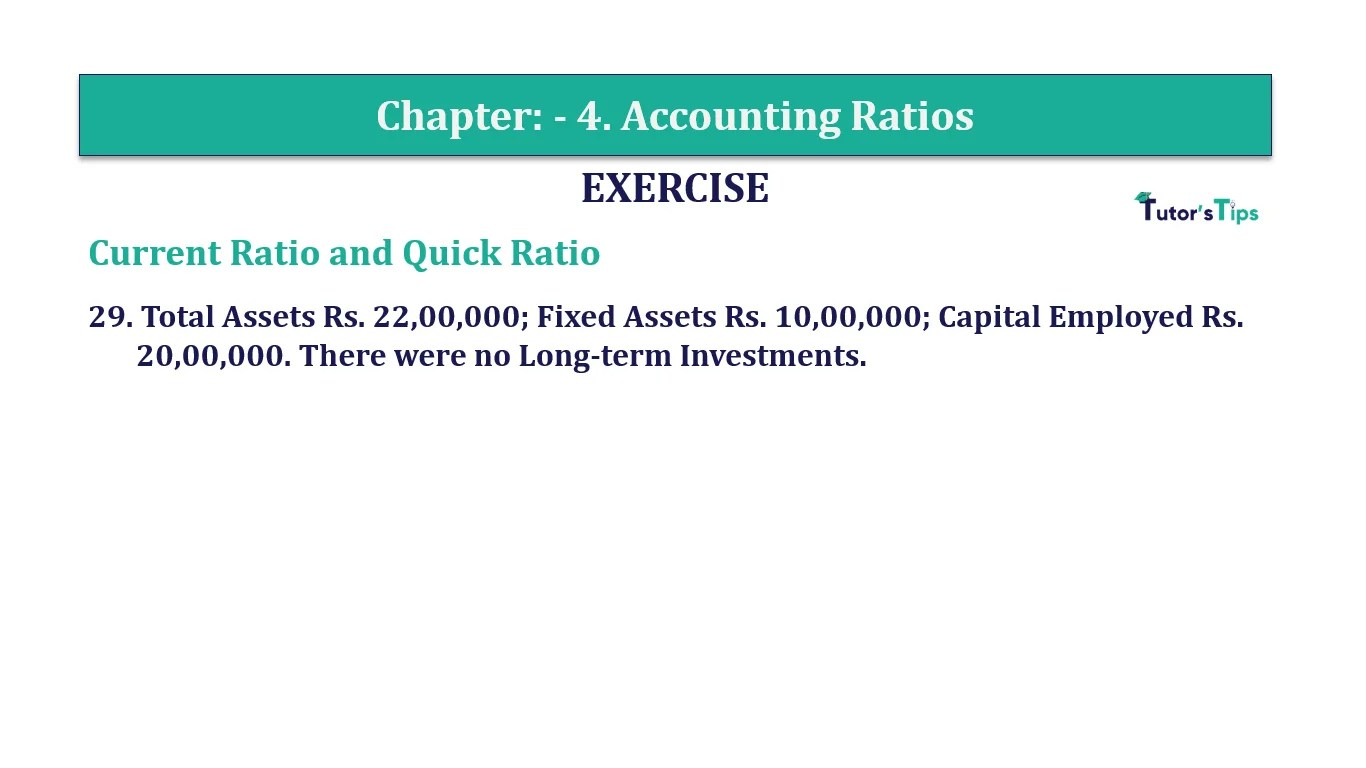# Question 29 Chapter 4 of +2-B – T.S. Grewal 12 ClassQuestion No. 29 - Chapter No.4 - T.S. Grewal +2 Book Part B

Question 29 Chapter 4 of +2-B

29. Total Assets Rs. 22,00,000; Fixed Assets Rs. 10,00,000; Capital Employed Rs.20,00,000. There were no Long-term Investments.

### The solution of Question 29 Chapter 4 of +2-B: –

 Fixed Assets = Rs. 10,00,000 Current Assets = Rs. 22,00,000 Current Assets = Total Assets – Fixed Assets Current Assets = Rs. 22,00,000 – Rs. 10,00,000 Current Assets = Rs. 12,00,000 Current liabilities = Rs. 6,00,000 Current liabilities = Total Assets + Capital Employed = Rs. 22,00,000 + Rs. 20,00,000 = Rs. 2,00,000

 Current Ratio = Current Assets = Rs.12,00,000 Current Liabilities Rs.2,00,000 = 6: 1

Balance Sheet: Meaning, Format & Examples

Comment if you have any question.

Also, Check out the solved question of previous Chapters: –